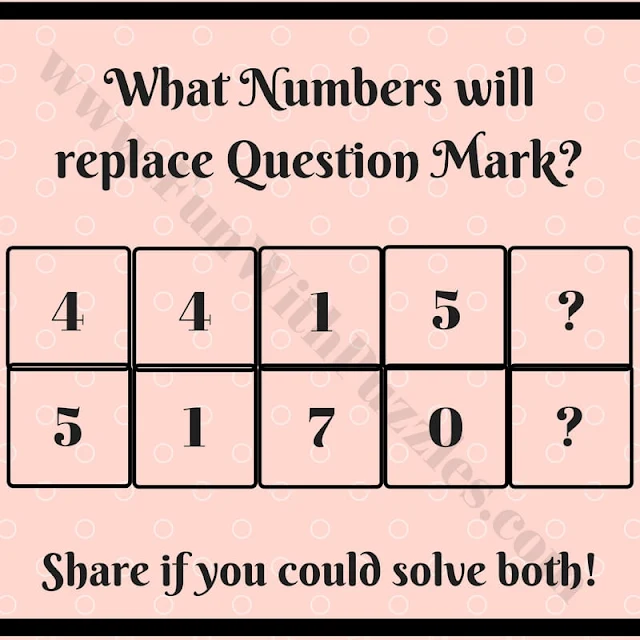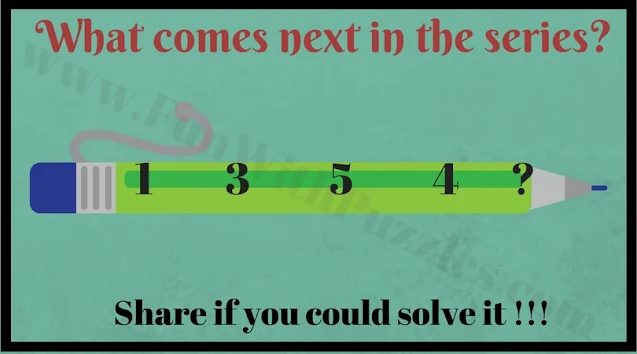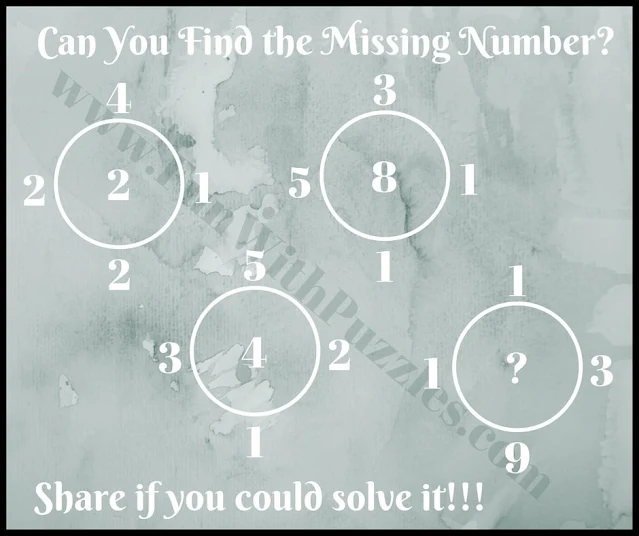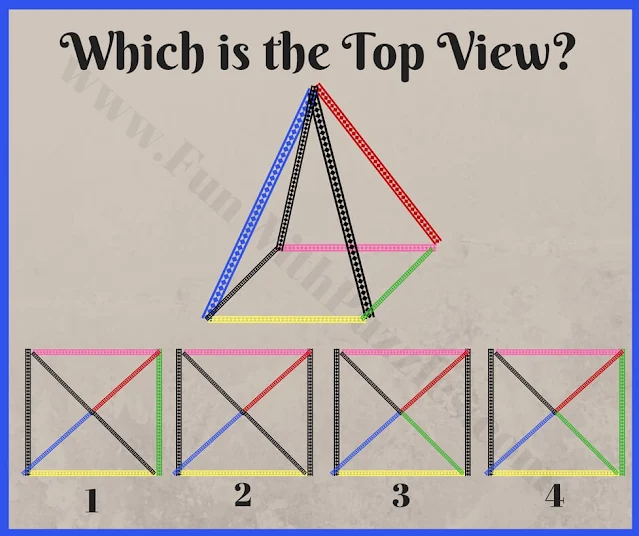Challenge yourself with the Ultimate IQ Test, a captivating collection of brain teasers that will push your cognitive abilities to the limit. This test is designed to assess your proficiency in Mathematics, Logic, and Visual Observation. Each brain teaser presents a unique puzzle, requiring you to think critically, apply mathematical calculations, and use logical reasoning to deduce the missing numbers and patterns represented by question marks.

In addition to testing your mathematical and logical acumen, the IQ Test also includes an intriguing Visual Brain Teaser. Here, you must engage your spatial intelligence to decipher the projection of a pyramid from a top view. Prepare to be captivated by the diverse range of challenges that await you in this comprehensive IQ assessment.

Are you up for the challenge? Solve each riddle with precision and clarity, and share your solutions and explanations in the comment section. Take this opportunity to enhance your problem-solving skills, sharpen your mind, and revel in the satisfaction of cracking these stimulating brain teasers.1. Tough math mind game brain teaser2. What is the next number in the sequence?3. Tough math brain riddle4. Easy logical deduction puzzle5. Non-verbal puzzle to find the top view of the Pyramid

This IQ Brain Test contained puzzles of different types like Mathematical, Logical, and Visual Picture Puzzles. Do check below mentioned Puzzles and Brain Teasers to challenge your brain.

## List of Puzzles, Brain Teasers, and Picture Puzzles

1) Brain Maths Logic Puzzles with Answers for Teens: It contains the logical puzzles in which some Maths Equations are given which are logically correct. Your challenge is to find the logical reasoning behind these Maths Equations and then solve the last equation.

2) Cool Mathematical Brain Teasers with answers: These are very interesting Maths Picture Brain Teasers in which some numbers are given in and around the square and your challenge is to find the missing number which will replace the question mark.

4) Mathematics Brain Test for Teens: At last, it contains the Maths Brain Test in which your challenge is to put the numbers at the sides of the triangles so that the addition of the numbers on each side of the triangle is the same.

## Answers to Brain Teasers and Riddles IQ Test

A1. 2 (top) and 5 (down)

A2. 4 (Four)

A3. 16

A4. 89

A5. 1

Unknown said...

I need more explanation on question number 1. How do we arrive at 2 and 5

Rajesh Kumar said...

Square both upper and lower number individually and add the result to get the number for the next card written from top to bottom.

Manoj Kumar said...

I need explanation on question number 2.

Rajesh Kumar said...

For the 2nd #brainteaser the logical reasoning used is as below
The next number in the sequence represents the number of letter in the previous number when that number is written in English e.g.
ONE-> Three letter and so the next number is 3.
Thus the sequence is ONE THREE FIVE FOUR FOUR and so on.

Manoj Kumar said...

Thank you @Rajesh

Unknown said...

thank you rajesh kumar.to give us wounderful answer.i want to know question no3 answer.

Unknown said...

need explanation on question number 3

Rajesh Kumar said...

Logical reasoning is explained as below for question number 3.
The number in the center of the Circle is the Multiplication of the Absolute Differences between the outer horizontal and vertical numbers.
So in the last case the central number is (9-1) * (2-1) = 16.
Please let me know if this logical reasoning is not clear for this question?

Unknown said...

Please explain again question no 1 with detail

Unknown said...

Please give me detail explanation of question 1